International Tables for Crystallography (2006). Vol. D, ch. 1.5, pp. 105-149https://doi.org/10.1107/97809553602060000632

Contents

• 1.5. Magnetic properties  (pp. 105-149)
• 1.5.1. Introduction  (pp. 105-109) | html | pdf |
• 1.5.1.1. Disordered magnetics  (pp. 106-107) | html | pdf |
• 1.5.1.2. Ordered magnetics  (pp. 107-109) | html | pdf |
• 1.5.1.2.1. Ferromagnets (including ferrimagnets)  (pp. 107-108) | html | pdf |
• 1.5.1.2.2. Antiferromagnets  (p. 108) | html | pdf |
• 1.5.1.2.3. Helical and sinusoidal magnetics  (pp. 108-109) | html | pdf |
• 1.5.2. Magnetic symmetry  (pp. 109-116) | html | pdf |
• 1.5.2.1. Magnetic point groups  (pp. 109-112) | html | pdf |
• 1.5.2.2. Magnetic lattices  (pp. 112-115) | html | pdf |
• 1.5.2.3. Magnetic space groups  (pp. 115-116) | html | pdf |
• 1.5.2.4. Exchange symmetry  (p. 116) | html | pdf |
• 1.5.3. Phase transitions into a magnetically ordered state  (pp. 116-125) | html | pdf |
• 1.5.3.1. Magnetic structures in rhombohedral crystals  (pp. 117-118) | html | pdf |
• 1.5.3.2. Exchange and magnetic anisotropy energies  (pp. 118-120) | html | pdf |
• 1.5.3.3. The thermodynamic theory of transitions into a magnetically ordered state  (pp. 120-125) | html | pdf |
• 1.5.3.3.1. Uniaxial ferromagnet  (p. 123) | html | pdf |
• 1.5.3.3.2. Uniaxial antiferromagnet  (pp. 124-125) | html | pdf |
• 1.5.4. Domain structure  (pp. 125-127) | html | pdf |
• 1.5.4.1. 180° domains  (pp. 125-126) | html | pdf |
• 1.5.4.2. Twin domains  (pp. 126-127) | html | pdf |
• 1.5.4.3. Ferroic domains  (p. 127) | html | pdf |
• 1.5.5. Weakly non-collinear magnetic structures  (pp. 127-131) | html | pdf |
• 1.5.5.1. Weak ferromagnetism  (pp. 127-130) | html | pdf |
• 1.5.5.2. Other weakly non-collinear magnetic structures  (pp. 130-131) | html | pdf |
• 1.5.6. Reorientation transitions  (pp. 131-132) | html | pdf |
• 1.5.7. Piezomagnetism  (pp. 132-137) | html | pdf |
• 1.5.7.1. Piezomagnetic effect  (pp. 132-136) | html | pdf |
• 1.5.7.2. Linear magnetostriction  (p. 136) | html | pdf |
• 1.5.7.3. Linear magnetic birefringence  (p. 137) | html | pdf |
• 1.5.8. Magnetoelectric effect  (pp. 137-142) | html | pdf |
• 1.5.8.1. Linear magnetoelectric effect  (pp. 138-140) | html | pdf |
• 1.5.8.2. Nonlinear magnetoelectric effects  (pp. 140-141) | html | pdf |
• 1.5.8.3. Ferromagnetic and antiferromagnetic ferroelectrics  (pp. 141-142) | html | pdf |
• 1.5.9. Magnetostriction  (pp. 142-146) | html | pdf |
• 1.5.9.1. Spontaneous magnetostriction  (pp. 143-144) | html | pdf |
• 1.5.9.2. Magnetostriction in an external magnetic field  (pp. 144-145) | html | pdf |
• 1.5.9.3. The difference between the magnetic anisotropies at zero strain and zero stress  (pp. 145-146) | html | pdf |
• 1.5.10. Transformation from Gaussian to SI units  (p. 146) | html | pdf |
• 1.5.11. Glossary  (p. 146) | html | pdf |
• References | html | pdf |
• Figures
• Fig. 1.5.1.1. Temperature dependence of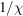at high temperatures for different types of magnetics  (p. 106) | html | pdf |
• Fig. 1.5.1.2. Ordered arrangements of magnetic moments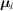in: ( a ) an ordinary ferromagnet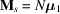; ( b ) a ferrimagnet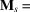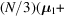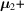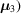; ( c ) a weak ferromagnet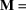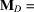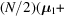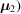,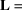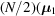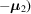, (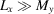;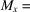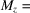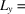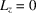)  (p. 107) | html | pdf |
• Fig. 1.5.1.3. Ordered arrangements of magnetic momentsin: ( a ) an ordinary two-sublattice antiferromagnet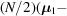; ( b ) a weakly non-collinear four-sublattice antiferromagnet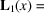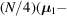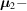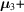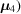,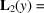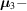; ( c ) a strongly non-collinear three-sublattice antiferromagnet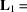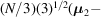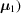,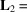(p. 108) | html | pdf |
• Fig. 1.5.1.4. Helical and sinusoidal magnetic structures  (p. 109) | html | pdf |
• Fig. 1.5.2.1. Magnetic lattices of the triclinic system  (p. 113) | html | pdf |
• Fig. 1.5.2.2. Magnetic lattices of the monoclinic system  (p. 113) | html | pdf |
• Fig. 1.5.2.3. Magnetic lattices of the orthorhombic system  (p. 114) | html | pdf |
• Fig. 1.5.2.4. Magnetic lattices of the tetragonal system  (p. 115) | html | pdf |
• Fig. 1.5.2.5. Magnetic lattices of the rhombohedral system  (p. 115) | html | pdf |
• Fig. 1.5.2.6. Magnetic lattices of the hexagonal system  (p. 115) | html | pdf |
• Fig. 1.5.2.7. Magnetic lattices of the cubic system  (p. 116) | html | pdf |
• Fig. 1.5.3.1. Arrangement of the symmetry elements of the group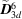(p. 117) | html | pdf |
• Fig. 1.5.3.2. Crystallographic structure of transition-metal oxides of the type-Fe 2 O 3   (p. 117) | html | pdf |
• Fig. 1.5.3.3. Crystallographic structure of transition-metal carbonates of the type MnCO 3   (p. 117) | html | pdf |
• Fig. 1.5.3.4. Four types of magnetic structures of rhombohedral oxides of transition metals  (p. 118) | html | pdf |
• Fig. 1.5.3.5. The conventional unit cell of UO 2   (p. 121) | html | pdf |
• Fig. 1.5.3.6. Temperature dependence of the mass susceptibility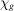for a uniaxial antiferromagnet along (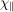) and perpendicular (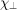) to the axis of antiferromagnetism  (p. 124) | html | pdf |
• Fig. 1.5.3.7. Dependence of the relative magnetization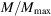on the magnetic field at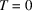(p. 124) | html | pdf |
• Fig. 1.5.3.8. Magnetic phase diagram for a uniaxial antiferromagnet in a magnetic field applied parallel to the axis  (p. 125) | html | pdf |
• Fig. 1.5.3.9. Phase diagram for a uniaxial antiferromagnet in the proximity of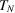, calculated for MnCl 2 ·4H 2 O  (p. 125) | html | pdf |
• Fig. 1.5.4.1. Magnetization curves of hexagonal cobalt  (p. 126) | html | pdf |
• Fig. 1.5.4.2. Magnetization curves of two cubic crystals (iron and nickel) for three crystallographic directions  (p. 126) | html | pdf |
• Fig. 1.5.4.3. Schematic display of the magnetization: ( a ) along the easy axis; ( b ) at an arbitrary angle to the easy axis; ( c ) perpendicular to the easy axis  (p. 126) | html | pdf |
• Fig. 1.5.4.4. A 180° domain wall in an antiferromagnet  (p. 126) | html | pdf |
• Fig. 1.5.5.1. Diagrams demonstrating two weakly ferromagnetic structures in rhombohedral crystals with two magnetic ions in the primitive cell  (p. 128) | html | pdf |
• Fig. 1.5.5.2. Dependence of magnetization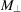and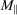on the magnetic field H for the weak ferromagnet MnCO 3 at 4.2 K  (p. 128) | html | pdf |
• Fig. 1.5.5.3. Magnetic structures of fluorides of transition metals  (p. 128) | html | pdf |
• Fig. 1.5.5.4. Magnetic structures of orthoferrites and orthochromites RM O 3   (p. 129) | html | pdf |
• Fig. 1.5.5.5. Temperature dependence of the susceptibility for CoCO 3   (p. 130) | html | pdf |
• Fig. 1.5.5.6. A weakly non-collinear magnetic structure corresponding to (1.5.5.12)  (p. 130) | html | pdf |
• Fig. 1.5.6.1. Schematic representation of the rotation of the vectors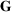and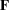(in the xz plane) at a reorientation transition in orthoferrites  (p. 131) | html | pdf |
• Fig. 1.5.6.2. Schematic representation of the rotation of the vector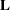under the action of a magnetic field applied to CoF 2 perpendicular to the fourfold axis z (reorientation transition)  (p. 132) | html | pdf |
• Fig. 1.5.7.1. The dependence of the magnetic moment of CoF 2 on the magnetic field  (p. 135) | html | pdf |
• Fig. 1.5.7.2. Linear magnetostriction of CoF 2   (p. 136) | html | pdf |
• Fig. 1.5.7.3. Variation of symmetry of the crystal field in the presence of the piezomagnetic effect in CoF 2   (p. 137) | html | pdf |
• Fig. 1.5.8.1. Temperature dependence of the components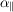and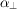in Cr 2 O 3   (p. 139) | html | pdf |
• Fig. 1.5.8.2. The hysteresis loop in the linear magnetoelectric effect in ferromagnetoelectric Ni 3 B 7 O 13 I at 46 K  (p. 142) | html | pdf |
• Fig. 1.5.9.1. Diagram explaining the occurrence of magnetostrictive strains in the demagnetized and saturated states of a cube-shaped crystal with a cubic prototype  (p. 144) | html | pdf |
• Tables
• Table 1.5.2.1. Comparison of different symbols for magnetic point groups  (p. 109) | html | pdf |
• Table 1.5.2.2. Comparison of different symbols for the elements of magnetic point groups  (p. 109) | html | pdf |
• Table 1.5.2.3. The 90 magnetic point groups of types 2 and 3  (pp. 110-111) | html | pdf |
• Table 1.5.2.4. List of the magnetic classes in which ferromagnetism is admitted  (p. 112) | html | pdf |
• Table 1.5.3.1. Two types of symbols for collinear antiferromagnetic and ferromagnetic structures  (p. 118) | html | pdf |
• Table 1.5.3.2. Sign variation of the components of antiferromagnetic and ferromagnetic vectors during transformations of the groupin rhombohedral crystals with four magnetic ions  (p. 119) | html | pdf |
• Table 1.5.3.3. Magnetic groups of symmetry in rhombohedral oxides of trivalent transition-metal ions  (p. 119) | html | pdf |
• Table 1.5.3.4. Magnetic point groups in rhombohedral oxides of transition metals  (p. 119) | html | pdf |
• Table 1.5.3.5. The signs of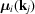for four sitesof the conventional unit cell (the corners of a primitive cell)  (p. 121) | html | pdf |
• Table 1.5.3.6. Characters of the irreducible representations of the group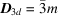and corresponding magnetic structures  (p. 122) | html | pdf |
• Table 1.5.5.1. The numbers of the crystallographic space groups that allow a phase transition into a weakly ferromagnetic state and the invariants of lowest order that are responsible for weak ferromagnetism  (p. 129) | html | pdf |
• Table 1.5.5.2. Magnetic point groups that allow weak ferromagnetism  (p. 130) | html | pdf |
• Table 1.5.7.1. The forms of the matrix characterizing the piezomagnetic effect  (p. 133) | html | pdf |
• Table 1.5.7.2. Experimental data for the piezomagnetic effect (PM) and for linear magnetostriction (LM)  (p. 136) | html | pdf |
• Table 1.5.8.1. The forms of the tensor characterizing the linear magnetoelectric effect  (p. 138) | html | pdf |
• Table 1.5.8.2. A list of some magnetoelectrics  (p. 139) | html | pdf |
• Table 1.5.8.3. Classification of the 122 magnetic point groups according to magnetoelectric types  (p. 140) | html | pdf |
• Table 1.5.8.4. List of the magnetic point groups of the ferromagnetoelectrics  (p. 141) | html | pdf |
• Table 1.5.9.1. Correspondence between matrix indices, A and tensor indices of the tensors describing spontaneous magnetostriction  (p. 144) | html | pdf |
• Table 1.5.9.2. Magnetostriction data for ferromagnets with prototype symmetry(p. 145) | html | pdf |
• Table 1.5.10.1. Conversion of Gaussian to SI units  (p. 146) | html | pdf |